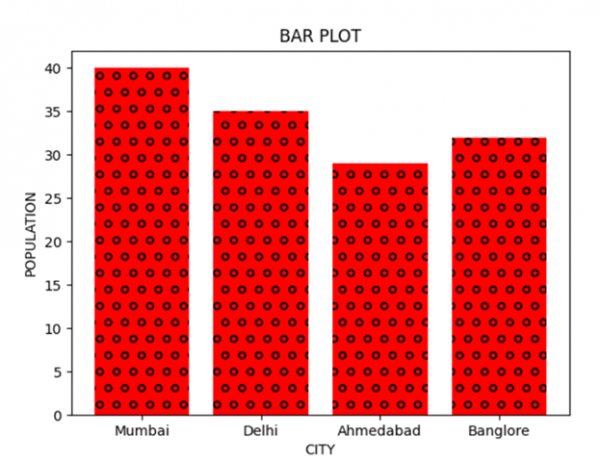# Adding textures to graphs using Matplotlib

In this program, we will plot a bar graph using the matplotlib library. The most important Step in solving matplotlib related problems using the matplotlib library is importing the matplotlib library. The syntax is:

import matplotlib.pyplot as plt

Pyplot is a collection of command style functions that make Matplotlib work like MATLAB. In addition to plotting the bar graphs, we will also add some textures to the graphs. The 'hatch' parameter in the bar() function is used to define the texture of the bar

## Algorithm

Step 1: Define a list of values.
Step 2: Use the bar() function and define parameters like xaxis, yaxis, hatch.
Step 3: Plot the graph.

## Example Code

import matplotlib.pyplot as plt

data_x = ['Mumbai', 'Delhi', 'Ahmedabad', 'Banglore']
data_y = [40, 35, 29, 32]

plt.xlabel("CITY")
plt.ylabel("POPULATION")
plt.title("BAR PLOT")
plt.bar(data_x, data_y, color='red', hatch = 'o')
plt.show()

## Output